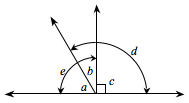### Home > MC2 > Chapter 8 > Lesson 8.2.1 > Problem8-35

8-35.Copy the diagram at right on your paper. Then use the diagram to answer the questions below.

1. Name two pairs of angles that are supplementary.

Supplementary angles add up to $180°$.

Look at angle $d$.
What other angle can you add to that to make a sum of $180°$?
What can you add to angle $c$ to get $180°$?

2. Name one pair of complementary angles.

Complementary angles add up to $90°$.

Which angle can you add to angle $a$ to make a sum of $90°$?

3. Name two right angles.

One right angle is angle $e$.
Which is the other right angle?

4. Name one obtuse angle.

Which is the only angle that is greater than $90°$?